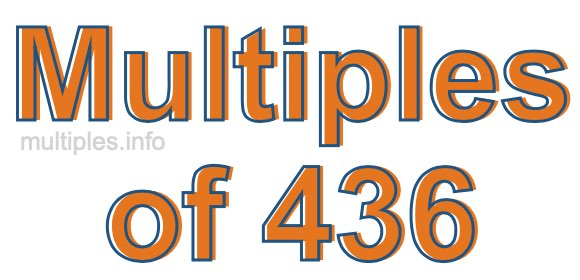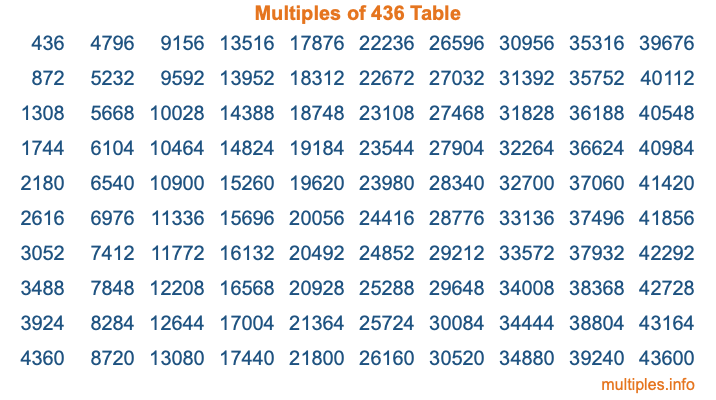Multiples of 436Welcome to the Multiples of 436 page. Here we will first teach you everything you will ever need to know about the multiples of 436, and then give you a study guide summary of everything we taught you to make sure you remember it all. Use this page to look up facts and learn information about the multiples of 436. This page will make you a multiples of four hundred thirty-six expert!

Definition of Multiples of 436
Multiples of 436 are all the numbers that when divided by 436 equal an integer. Each of the multiples of 436 are called a multiple. A multiple of 436 is created by multiplying 436 by an integer.

Therefore, to create a list of multiples of 436, you start with 1 multiplied by 436, then 2 multiplied by 436, then 3 multiplied by 436, and so on for as long as you want. Thus, the list of the first five multiples of 436 is 436, 872, 1308, 1744, and 2180. To see a larger list of multiples of 436, see the printable image of Multiples of 436 further down on this page. We also have a category where you can choose any nth multiple of 436.

Multiples of 436 Checker
The Multiples of 436 Checker below checks to see if any number of your choice is a multiple of 436. In other words, it checks to see if there is any number (integer) that when multiplied by 436 will equal your number. To do that, we divide your number by 436. If the the quotient is an integer, then your number is a multiple of 436.

Is  a multiple of 436?

Least Common Multiple of 436 and ...
A Least Common Multiple (LCM) is the lowest multiple that two or more numbers have in common. This is also called the smallest common multiple or lowest common multiple and is useful to know when you are adding our subtracting fractions. Enter one or more numbers below (436 is already entered) to find the LCM.

Check out our LCM Calculator if you need more details about the Least Common Multiple or if you need the LCM for different numbers for adding and subtraction fractions.

nth Multiple of 436
As we stated above, 436 is the first multiple of 436, 872 is the second multiple of 436, 1308 is the third multiple of 436, and so on. Enter a number below to find the nth multiple of 436.

th multiple of 436

Multiples of 436 vs Factors of 436
436 is a multiple of 436 and a factor of 436, but that is where the similarities end. All postive multiples of 436 are 436 or greater than 436. All positive factors of 436 are 436 or less than 436.

Below is the beginning list of multiples of 436 and the factors of 436 so you can compare:

Multiples of 436: 436, 872, 1308, 1744, 2180, etc.

Factors of 436: 1, 2, 4, 109, 218, 436

As you can see, the multiples of 436 are all the numbers that you can divide by 436 to get a whole number. The factors of 436, on the other hand, are all the whole numbers that you can multiply by another whole number to get 436.

It's also interesting to note that if a number (x) is a factor of 436, then 436 will also be a multiple of that number (x).

Multiples of 436 vs Divisors of 436
The divisors of 436 are all the integers that 436 can be divided by evenly. Below is a list of the divisors of 436.

Divisors of 436: 1, 2, 4, 109, 218, 436

The interesting thing to note here is that if you take any multiple of 436 and divide it by a divisor of 436, you will see that the quotient is an integer.

Multiples of 436 Table
Below is an image of the first 100 multiples of 436 in a table. The table is in chronological order, column by column. The first column has the first ten multiples of 436, the second column has the next ten multiples of 436, and so on.The Multiples of 436 Table is also referred to as the 436 Times Table or Times Table of 436. You are welcome to print out our table for your studies.

Negative Multiples of 436
Although not often discussed or needed in math, it is worth mentioning that you can make a list of negative multiples of 436 by multiplying 436 by -1, then by -2, then by -3, and so on, to get the following list of negative multiples of 436:

-436, -872, -1308, -1744, -2180, etc.

Multiples of 436 Summary
Below is a summary of important Multiples of 436 facts that we have discussed on this page. To retain the knowledge on this page, we recommend that you read through the summary and explain to yourself or a study partner why they hold true.

There are an infinite number of multiples of 436.

A multiple of 436 divided by 436 will equal a whole number.

436 divided by a factor of 436 equals a divisor of 436.

The nth multiple of 436 is n times 436.

The largest factor of 436 is equal to the first positive multiple of 436.

436 is a multiple of every factor of 436.

436 is a multiple of 436.

A multiple of 436 divided by a divisor of 436 equals an integer.

436 divided by a divisor of 436 equals a factor of 436.

Any integer times 436 will equal a multiple of 436.

Multiples of a Number
Here you can get the multiples of another number, all with the same attention to detail as we did for multiples of 436 on this page.

Multiples of
Multiples of 437
Did you find our page about multiples of four hundred thirty-six educational? Do you want more knowledge? Check out the multiples of the next number on our list!